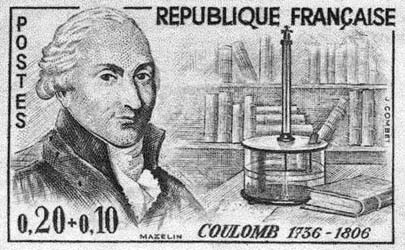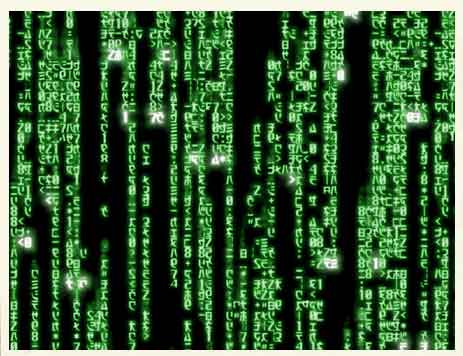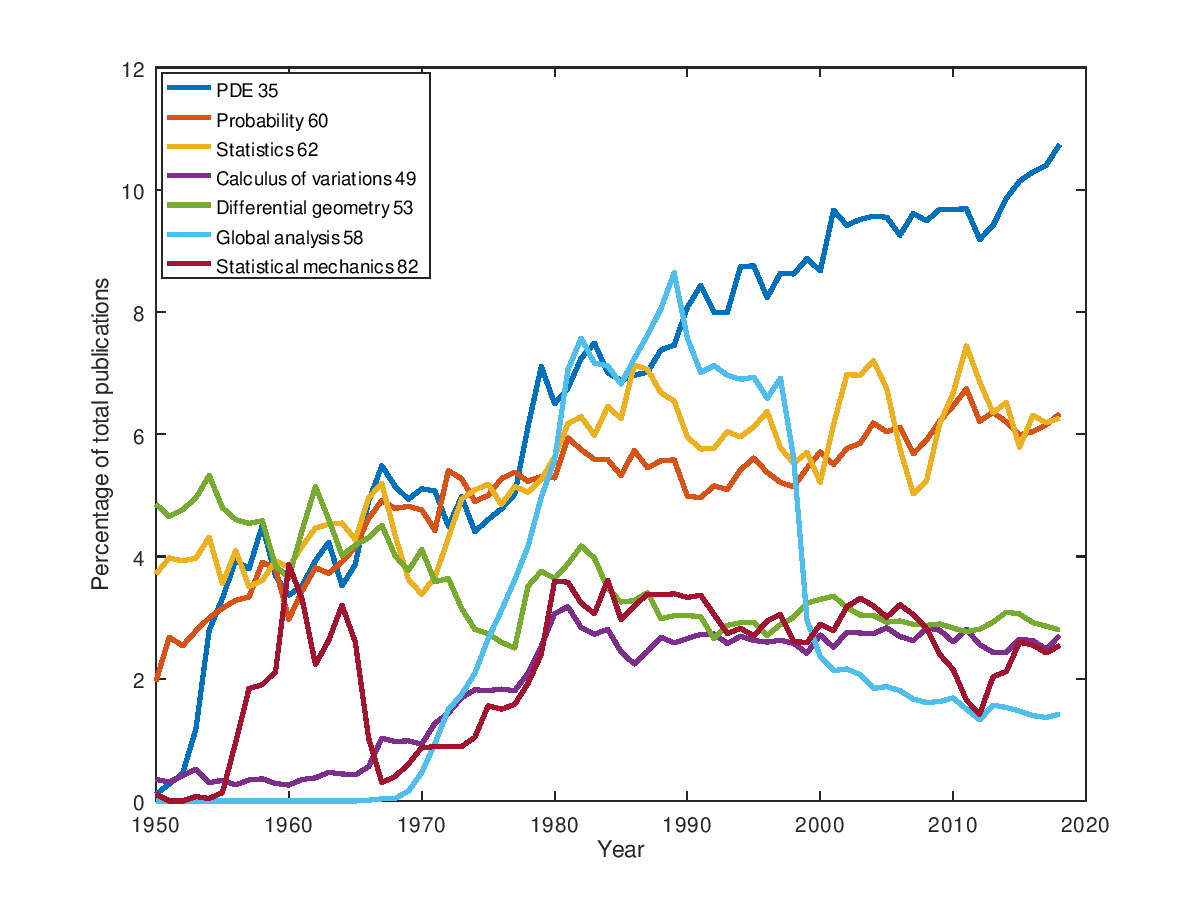Press "Enter" to skip to content

# Libres pensées d'un mathématicien ordinaire PostsAu sujet des modes de financement des publications académiques, les choses avancent, lentement, par à-coups. L’INSMI du CNRS a œuvré par exemple pour la création du Centre Mersenne à Grenoble. La montée en puissance et la conduite du changement ne sont pas simples. Idem pour le projet MathOA.  Plus récemment, sur le plan des actions macroscopiques structurantes, la France s’engage sur la science ouverte, tandis que l’Europe met en avant le diamond open access dans son Plan S pour 2021 !

La guerre continue sur d’autres fronts. En France, en mars 2019, le tribunal de grande instance de Paris a ordonné aux fournisseurs d’accès à Internet français de bloquer l’accès aux sites Sci-Hub et Library Genesis. La décision fait suite à une plainte déposée par Elsevier et Springer Nature. Mais ce blocage est contournable avec un simple VPN ou un serveur DNS alternatif. Cette décision de justice n’est qu’un  épisode de plus au long feuilleton judiciaire international. Toujours en France, Elsevier est aussi partie prenante d’un projet d’accord controversé avec le consortium Couperin.

En matière de publications, les universitaires sont à la fois les producteurs, les évaluateurs, et les consommateurs, au bout du compte rackettés et pris en otages par les mastodontes Elsevier et Springer. Le numérique n’a fait que forcer le trait. Il n’y a pas vraiment besoin d’être de gauche pour être contre la position de Elsevier et Springer: ils sont devenus des parasites, et il faudrait à minima les remettre à leur juste place de prestataires de services en concurrence.Charles-Augustin de Coulomb (1736-1806)

The Coulomb or Newton kernel in ${\mathbb{R}^d}$, ${d\geq1}$, is often defined for all ${x\neq0}$ as

$g(x):=\begin{cases} -|x| & \mbox{if }d=1,\\ \log\frac{1}{|x|} & \mbox{if }d=2,\\ \frac{1}{|x|^{d-2}} & \mbox{if }d\geq3, \end{cases}$

where ${|x|:=\sqrt{x_1^2+\cdots+x_d^2}}$ is the Euclidean norm. It is the fundamental solution of the Laplace or Poisson equation, in the sense that

$\Delta g=-c_d\delta_0 \quad\mbox{where}\quad c_d:= \begin{cases} 2 & \mbox{if }d=1,\\ 2\pi & \mbox{if }d=2,\\ (d-2)|\mathbb{S}^{d-1}| & \mbox{if }d\geq 3, \end{cases}$

where ${\mathbb{S}^{d-1}:=\{x\in\mathbb{R}^d:|x|=1\}}$, and, denoting ${\Gamma}$ the Euler Gamma function,

$|\mathbb{S}^{d-1}| =2\frac{\pi^{d/2}}{\Gamma(d/2)}.$

This partial differential equation is in the sense of Schwartz distributions ${\mathcal{D}'(\mathbb{R}^d)}$. Note that on ${\mathbb{R}^d\setminus\{0\}}$, the function ${g}$ is ${\mathcal{C}^\infty}$ and harmonic in the sense that ${\Delta g(x)=\partial_1^2g(0)+\cdots+\partial_d^2g(0)=0}$ as a function for all ${x\neq0}$. The behavior at the origin makes ${g}$ super-harmonic (its Laplacian is ${\leq0}$), which is a trace analogue of concavity.

The case ${d=1}$ is intuitive: the derivative of ${|x|}$ in the sense of distributions is the Heaviside function ${-\mathbf{1}_{x<0}+\mathbf{1}_{x>0}}$ (essentially a jump at zero of height ${2}$), and the second derivative twice the Dirac mass, ${2\delta_0}$. The case ${d=2}$ appears also as special: it blows up at infinity.

The physical interpretation of ${g(x)}$, up to physical constants, is the potential generated at point ${x}$ by a charge at the origin, the field being ${-\nabla g(x)}$. The electric field if we model electrostatics (Coulomb), and the gravitational field if we model gravity (Newton). Note that ${-g(x)\nabla g(x)}$ vanishes at infinity if ${d\geq2}$, but not if ${d=1}$, which makes a difference for integration by parts.

For a probability measure ${\mu}$ on ${\mathbb{R}^d}$, the potential generated by ${\mu}$ at point ${x}$ is

$U_\mu(x)=(g*\mu)(x)=\int g(x-y)\mu(\mathrm{d}y).$

In dimension ${d=1}$ or ${d=2}$, this is well defined as soon as ${\mu}$ integrates ${g}$ at infinity. Note that ${g}$ is Lebesgue locally integrable. The convolution operator ${\mu\mapsto U_\mu}$ is the inverse of the Laplacian, and we have the inversion formula

$\Delta U_\mu=(\Delta g)*\mu=-c_d(\delta_0*\mu)=-c_d\mu,$

in ${\mathcal{D}'(\mathbb{R}^d)}$. The potential ${U_\mu}$ is harmonic outside the support of ${\mu}$.

Alternative formulation. The following alternative definition is simpler:

$g(x):=\begin{cases} \frac{1}{(d-2)|x|^{d-2}} & \mbox{if }d=1\mbox{ or } d\geq3,\\ \log\frac{1}{|x|} & \mbox{if }d=2, \end{cases}$

which satisfies

$\Delta g=-c_d\delta_0 \quad\mbox{where}\quad c_d:=|\mathbb{S}^{d-1}|$

with the convention ${|\mathbb{S}^0|:=|\{-1,1\}|=2}$. Indeed if ${d=1}$ then ${\frac{1}{(d-2)|x|^{d-2}}=-|x|}$.

This alternative formulation makes the equilibrium measure nicer in the quadratic confinement case. Namely, the electrostatic energy of a distribution of charges modeled by a probability measure ${\mu}$ on ${\mathbb{R}^d}$ with external field generated by a potential ${V:\mathbb{R}^d\rightarrow\mathbb{R}}$ is

$\mathcal{E}_V(\mu) :=\iint g(x-y)\mu(\mathrm{d}x)\mu(\mathrm{d}y)+\int V(x)\mathrm{d}\mu(x).$

This functional is strictly convex and lower semi-continuous with respect to the narrow convergence of probability measures. Its minimizer, ${\mu_*=\arg\inf\mathcal{E}_V}$, is called the equilibrium measure. The Euler-Lagrange equation gives that ${\mu_*}$ has density

$\frac{\Delta V}{2c_d}$

on its support. When ${V}$ is strong enough at infinity then this support is compact. For instance when ${V(x)=|x|^2}$ then we find that the equilibrium measure ${\mu_*}$ is the uniform distribution on the unit ball of ${\mathbb{R}^d}$. Indeed, in this case

$\frac{\Delta V}{2c_d} =\frac{2d}{2|\mathbb{S}^{d-1}|} =\frac{2d}{2d|\mathbb{B}^d|} =\frac{1}{|\mathbb{B}^d|}$

where ${\mathbb{B}^d=\{x\in\mathbb{R}^d:|x|=1\}}$ is the unit ball of ${\mathbb{R}^d}$. Recall that if ${s(r)}$ and ${v(r)}$ are respectively the surface of the sphere of radius ${r}$ and the volume of the ball of radius ${r}$ in ${\mathbb{R}^d}$ then ${v(r)=r^dv(1)}$ and ${s(r)=v'(r)=dr^{d-1}v(1)}$ hence ${s(1)=dv(1)}$.

An even more compact definition. If we think the dimension ${d}$ as being a real positive number, we may observe that for all ${x\neq0}$,

$\lim_{d\rightarrow2}\frac{\frac{1}{|x|^{d-2}}-1}{d-2} =\partial_{s=0}\frac{1}{|x|^{s}} =\partial_{s=0}\mathrm{e}^{-s\log|x|} =-\log|x|.$

This means that the formula ${\frac{1}{(d-2)|x|^{d-2}}}$, already valid for ${d=1}$ and ${d\geq3}$, is actually also valid for ${d=2}$ provided that we remove a singularity. This suggests to define the kernel, for all integers ${d\geq1}$ and even all real numbers ${d\geq1}$, and all ${x\neq0}$, as

$g(x):=\frac{1}{(d-2)|x|^{d-2}}-\frac{1}{d-2},$

which satisfies

$\Delta g=-2\frac{\pi^{d/2}}{\Gamma(d/2)}\delta_0.$

Note that physically, the potential is important only for defining the field, and as a consequence, the potential is defined up to an additive constant. In other words, what matters is the differences of potential values rather than the values themselves.

Riesz kernels. The Riesz kernel in dimension ${d\geq2}$ and parameter ${s\geq0}$ with ${0<s<d}$ is defined for all ${x\neq0}$ by the formula

$g(x):=\frac{1}{|x|^{d-s}}.$

We recover the Coulomb/Newton kernel when ${s=2}$. The Riesz kernel is the fundamental solution of the fractional Laplacian, which is a Fourier multiplier, and a non-local operator when ${d\neq2}$. Its inverse is a convolution operator.

Conclusion. All in all, if we would like to incorporate all cases in a compact formula, we could consider in ${\mathbb{R}^d}$, ${d\geq1}$, for all ${s\in\mathbb{R}}$ and ${x\neq0}$ the kernel

$g(x):=\frac{1}{s|x|^s},$

with the convention ${g(x)=-\log|x|}$ if ${s=0}$. The Coulomb/Newton case corresponds to taking ${s=d-2}$ as we have seen. Indeed, this is more or less the choice often made by my colleague Mathieu Lewin for instance, see for example arXiv:1905.09138.

Entropy. The logarithm appears as a derivative of power functions also in relation with entropy and hypercontractivity, as explained in a previous post. The same for the derivation of the logarithmic Sobolev inequality from the Beckner inequalities.

Further reading.

Final word. Mathematics is also revealing common structures among apparently different things. In Physics, going beyond integers and the apparent physical meaning has advantages, as in the replica trick. You may already know this aphorism by Henri Bouasse, French Physicist from Toulouse of the 19-th century: Le physicien traite les problèmes du véhicule à une roue (la brouette), à deux roues (tilbury ou bicyclette), à trois, à quatre roues. Le mathématicien traite le problème général du véhicule à ${n}$ roues, ${n}$ étant entier ou fractionnaire, positif ou négatif, réel ou imaginaire.Trois idées basiques pour enseigner différemment :

• Demander au département d’installer un serveur JupyterHub pour faire du Julia/Python/R
• Utiliser le Google colaboratory ou rstudio.cloud pour faire du Python ou du R en ligne (1)
• Demander aux étudiants de rendre leur projet sur github pour apprendre à s’en servir (2)

(1) signalé par mon collègue Jamal Atif. L’analogue chez Microsoft est Azure Notebooks.

(2) signalé par mon collègue Robin Ryder.

Each journal article in MathSciNet is tagged with one or more MSC classification numbers. Here are the graphics for few of them around analysis, probability, and statistics (this is not exclusive since an article can contain multiple numbers).Same kind of graphics with more data (beware that the colors are not the same):Syntax · Style · Tracking & Privacy.